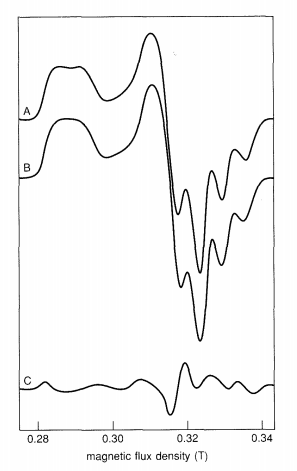# 8.2.5: Electron-transfer Rates

$$\newcommand{\vecs}{\overset { \rightharpoonup} {\mathbf{#1}} }$$ $$\newcommand{\vecd}{\overset{-\!-\!\rightharpoonup}{\vphantom{a}\smash {#1}}}$$$$\newcommand{\id}{\mathrm{id}}$$ $$\newcommand{\Span}{\mathrm{span}}$$ $$\newcommand{\kernel}{\mathrm{null}\,}$$ $$\newcommand{\range}{\mathrm{range}\,}$$ $$\newcommand{\RealPart}{\mathrm{Re}}$$ $$\newcommand{\ImaginaryPart}{\mathrm{Im}}$$ $$\newcommand{\Argument}{\mathrm{Arg}}$$ $$\newcommand{\norm}{\| #1 \|}$$ $$\newcommand{\inner}{\langle #1, #2 \rangle}$$ $$\newcommand{\Span}{\mathrm{span}}$$ $$\newcommand{\id}{\mathrm{id}}$$ $$\newcommand{\Span}{\mathrm{span}}$$ $$\newcommand{\kernel}{\mathrm{null}\,}$$ $$\newcommand{\range}{\mathrm{range}\,}$$ $$\newcommand{\RealPart}{\mathrm{Re}}$$ $$\newcommand{\ImaginaryPart}{\mathrm{Im}}$$ $$\newcommand{\Argument}{\mathrm{Arg}}$$ $$\newcommand{\norm}{\| #1 \|}$$ $$\newcommand{\inner}{\langle #1, #2 \rangle}$$ $$\newcommand{\Span}{\mathrm{span}}$$$$\newcommand{\AA}{\unicode[.8,0]{x212B}}$$

## Overview

Measurements of the rates of oxidation-reduction reactions began in the late 1940s. A great deal of the early experimental work was carried out by inorganic chemists, and by the 1970s the reactivity patterns of many complexes had been uncovered.49-51 Chemists studying the mechanisms of metalloprotein electron-transfer reactions frequently seek parallels with the redox behavior of less-complicated inorganic complexes.

In examining biological electron transfers, it is important to remember that metalloproteins are more than just metal ions in disguise. Virtually every property of a protein (excluding its amino-acid sequence) depends on the solution pH. Redox proteins are very large polyelectrolytes whose redox prosthetic groups are typically buried in the protein interior. One important distinction between redox reactions of proteins and redox reactions of small transition-metal complexes is the magnitude of the electron donor-to-acceptor distance. The relevant distance for small molecules, unlike redox proteins, is generally taken to be van der Waals contact. Within the last ten years, it has been convincingly demonstrated that electrons can "tunnel" at significant rates across distances of 15 Å or more in protein interiors.52-58

Experimental investigation of the factors that control the rates of biological redox reactions has not come as far as the study of the electron transfers of metal complexes, because many more variables must be dealt with (e.g., asymmetric surface charge, nonspherical shape, uncertain details of structures of proteins complexed with small molecules or other proteins). Many experimental approaches have been pursued, including the covalent attachment of redox reagents to the surfaces of metalloproteins.

## Self-exchange and Cross Reactions

The simplest reactions in solution chemistry are electron self-exchange reactions (Equation 6.10), in which the reactants and products are the same (the asterisk is used to identify a specific isotope).

$\;^{\ast}A_{ox} + A_{red} \rightarrow \;^{\ast}A_{red} + A_{ox} \tag{6.10}$

The only way to establish chemically that a reaction has taken place is to introduce an isotopic label. There is no change in the free energy ($$\Delta$$G° = 0) for this type of reaction. As will become evident later on, the reason why these types of reactions are studied is because self-exchange rates and activation parameters are needed to interpret redox reactions in which a net chemical change occurs. The experimental measurement58 of self-exchange rates is tedious and usually only results in an order-of-magnitude estimate of the rate constant (as inferred from the experimental timescale; see Table 6.2). Most of the protein self-exchange rates reported to date have been measured by NMR line-broadening studies. Other potentially useful methods, such as Mössbauer spectroscopy and EPR, have not been widely used.

### Table 6.2 - Experimental timescales in seconds

 Laser Flash Photolysis $$\geq 10^{-14}$$ Pulse Radiolysis $$\sim 10^{-9}$$ Mössbauer Spectroscopy (57Fe) $$10^{-9} - 10^{-6}$$ EPR (transition metals) $$10^{-9} - 10^{-8}$$ Temperature-jump Spectrometry $$\geq 10^{-8}$$ NMR (1H) $$\sim 10^{-5}$$ Chemical Mixing $$\geq 10^{-3}$$

An elegant example of the measurement of an electron self-exchange rate of a redox protein was reported by Dahlin et al.59 The copper ion of stellacyanin was removed and then replaced with either 63Cu or 65Cu. Oxidized [63Cu] stellacyanin was allowed to react with reduced [65Cu] stellacyanin for various times (10 ms to 7 min) at 20 °C, after which the reaction was quenched by lowering the solution temperature to -120°C using a rapid-freeze apparatus:

$\;^{63}Cu^{2+} + \;^{65}Cu^{+} \rightarrow \;^{63}Cu^{+} + \;^{65}Cu^{2+} \tag{6.11}$

Subtle differences in the EPR spectra (Figure 6.20) of the two isotopic forms of stellacyanin (due to a small difference in the nuclear magnetic moments of the two isotopes) were used to monitor the progress of the reaction, yielding a rate constant of 1.2 x 105 M-1s-1.Figure 6.20 - Frozen-solution EPR spectra of stellacyanin (20 K, 9.25 GHz): (A) 65Cu; (B) 63Cu; (C) Difference spectrum (A - B).59

Much more common are cross reactions (Equation 6.12), where Aox is the oxidized reactant, Bred is the reduced reactant, Ared is the reduced product, and Box is the oxidized product.

$A_{ox} + B_{red} \rightarrow A_{red} + B_{ox} \tag{6.12}$

For these reactions, $$\Delta$$G° $$\neq$$ 0. The experimental measurement of cross-reaction rates is generally more straightforward than the measurement of self-exchange rates. Either the reactants are simply mixed together, or a thermodynamically unstable system is generated rapidly (via pulse radiolysis, flash photolysis, or temperature-jump relaxation) to initiate the redox reaction. Absorption spectroscopy has almost always been used to monitor the progress of protein cross reactions. The primary goal of theory, as will become evident, is to provide a relationship between $$\Delta$$G° and $$\Delta$$G$$\ddagger$$ for cross reactions.

Both self-exchange and cross reactions can be broadly classified as inner-sphere or outer-sphere reactions. In an inner-sphere reaction, a ligand is shared between the oxidant and reductant in the transition state. An outer-sphere reaction, on the other hand, is one in which the inner coordination shells of both the oxidant and the reductant remain intact in the transition state. There is no bond breaking or bond making, and no shared ligands between redox centers. Long-range electron transfers in biology are all of the outer-sphere type.

8.2.5: Electron-transfer Rates is shared under a not declared license and was authored, remixed, and/or curated by LibreTexts.